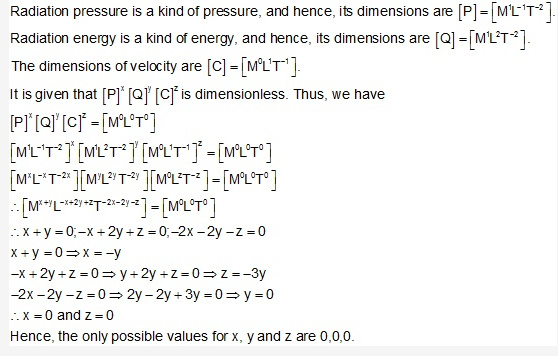# If P represents radiation pressure, C represents velocity of light

if P represents radiation pressure, C represents velocity of light and Q represents radiation striking per unit area per second, then non-zero integers x,y, and z are such that (Px)(Qy)(Cz) is dimensionless are ?